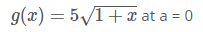# Find the linear approximation of the function g(x) = 5sqrt(1+x) at a = 0

Find the linear approximation of the functiong (x)= ?
Use it to approximate the numbers 5 √ .95 and 5 √ 1.1 . (Round your answers to three decimal places.)
5 √ .95 = ?
5 √ 1.1 =?